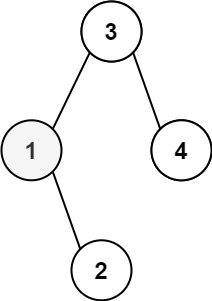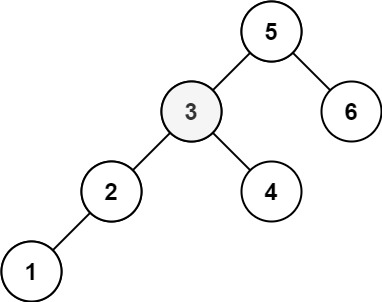| English | 简体中文 |

# 230. Kth Smallest Element in a BST

## Description

Given the root of a binary search tree, and an integer k, return the kth smallest value (1-indexed) of all the values of the nodes in the tree.

Example 1:Input: root = [3,1,4,null,2], k = 1
Output: 1


Example 2:Input: root = [5,3,6,2,4,null,null,1], k = 3
Output: 3


Constraints:

• The number of nodes in the tree is n.
• 1 <= k <= n <= 104
• 0 <= Node.val <= 104

Follow up: If the BST is modified often (i.e., we can do insert and delete operations) and you need to find the kth smallest frequently, how would you optimize?